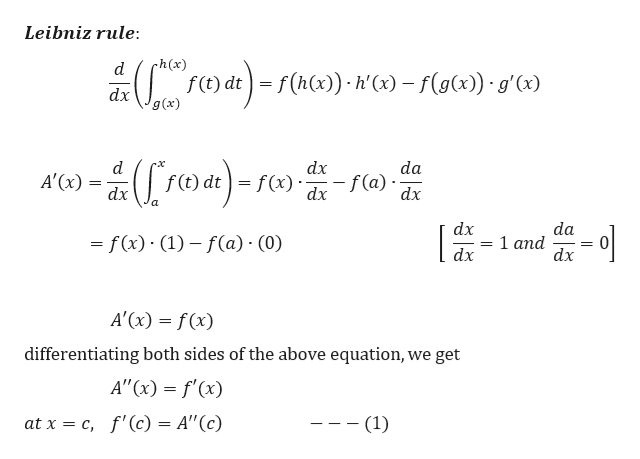Question
16 views
check_circle

Step 1

It is given that f is continuous and differentiable such that

Step 2

We know th...help_outlineImage TranscriptioncloseLeibniz rule: ch(x) d f(t) dt ) = f(h(x)) · h'(x) – f(g(x)) · g'(x) g(x) -ź([roa)-rc» -1 da d A'(x) dx dx - f(a) · dx dx dx da = f(x) · (1) – f(a) · (0) %3D dx dx A'(x) = f (x) differentiating both sides of the above equation, we get A"(x) = f'(x) at x = c, f'(c) = A"(c) -- (1) %3| fullscreen

### Want to see the full answer?

See Solution

#### Want to see this answer and more?

Solutions are written by subject experts who are available 24/7. Questions are typically answered within 1 hour.*

See Solution
*Response times may vary by subject and question.
Tagged in

### Calculus